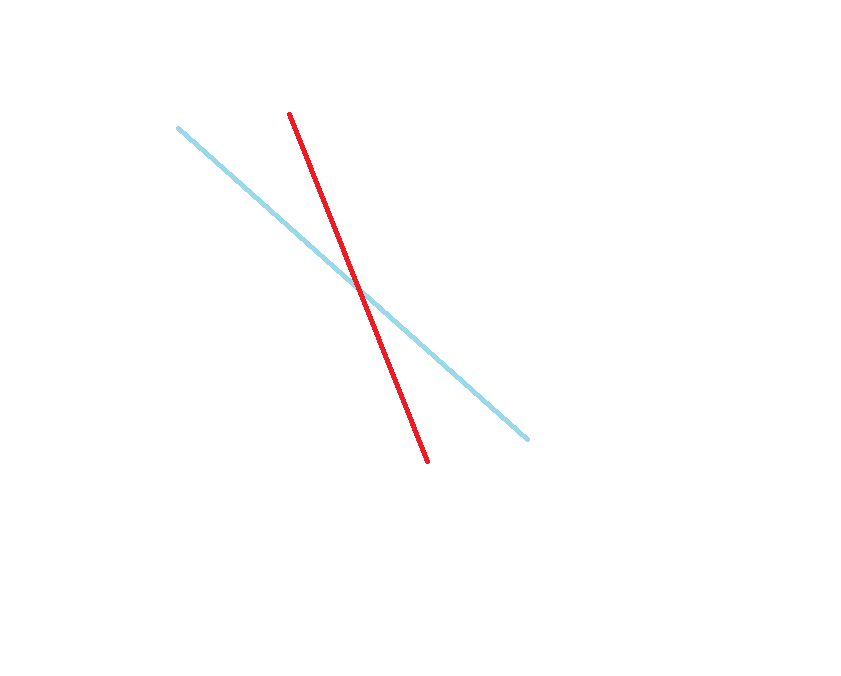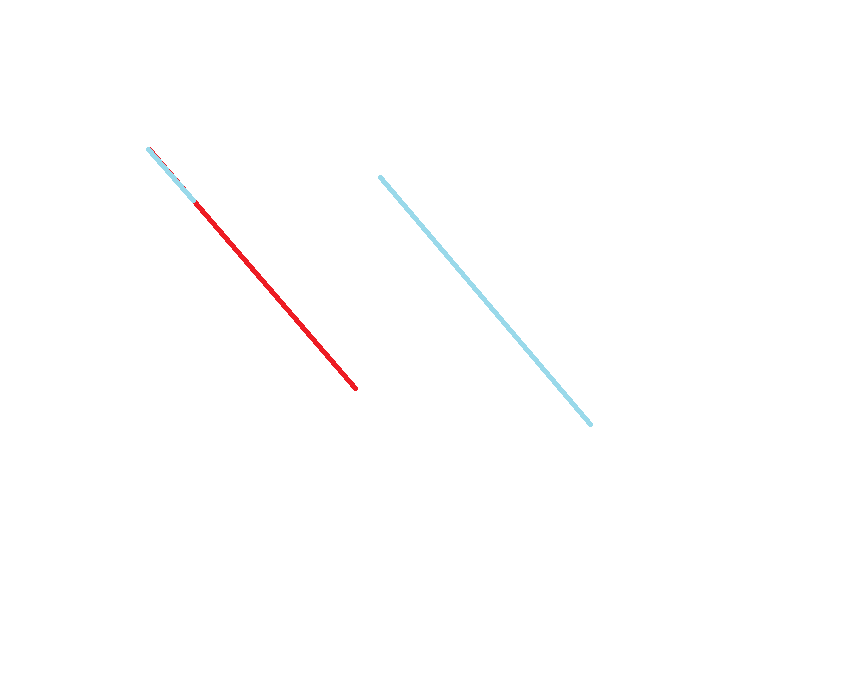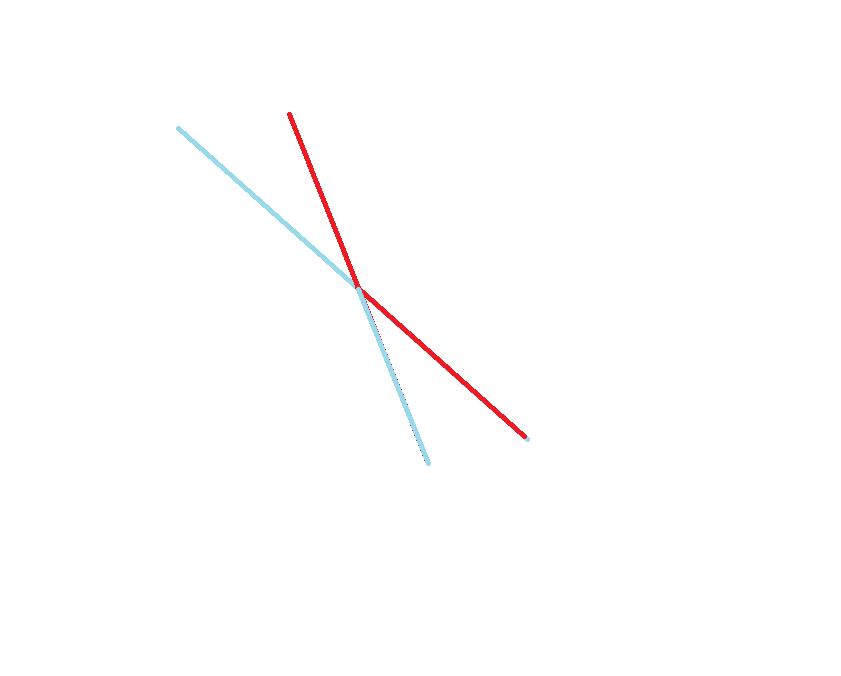## HDU - 1257

Input

Output

Sample Input
8 389 207 155 300 299 170 158 65
Sample Output
2

(拦截系统发射炮弹,敌国发射导弹袭击)``````#include <cstdio>
#include <set>
#include <iostream>
using namespace std;
multiset <int> s;
int main()
{
int n;
while (~scanf("%d",&n))
{
int ans=0;
s.clear();
for (int i=1;i<=n;i++)
{
int x;
scanf("%d",&x);
if (s.upper_bound(x)!=s.end()) s.erase(s.upper_bound(x));
else ans++;
s.insert(x);
}
printf("%d\n",ans);
}
}
``````